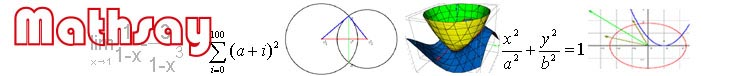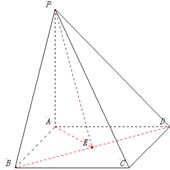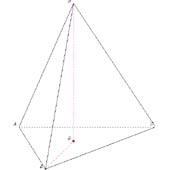Home

Support

Examples

Geometry

Trigonometry

Coordinate geometry

Function

Equation

Vector

3D geometry

3D plot

Limit & derivative

Math symbolRectangle ABCD, AB=20, AD=20. PA and plane ABCD are vertical each other. PA=20.  Calculate dihedral angle A-BD-P.Regular three-pyramid H-ABC. HG is vertical with ABC. Calculate the length of BG.